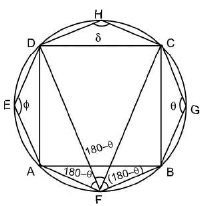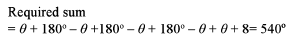Courses

# Test: Geometry- 1

## 15 Questions MCQ Test Quantitative Aptitude for SSC CGL | Test: Geometry- 1

Description
This mock test of Test: Geometry- 1 for Quant helps you for every Quant entrance exam. This contains 15 Multiple Choice Questions for Quant Test: Geometry- 1 (mcq) to study with solutions a complete question bank. The solved questions answers in this Test: Geometry- 1 quiz give you a good mix of easy questions and tough questions. Quant students definitely take this Test: Geometry- 1 exercise for a better result in the exam. You can find other Test: Geometry- 1 extra questions, long questions & short questions for Quant on EduRev as well by searching above.
QUESTION: 1

### In the given figure, MN and KL are parallel lines.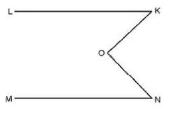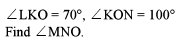Solution: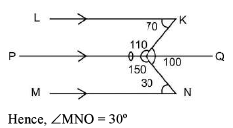QUESTION: 2

Solution:

x + 5x = 180°

6x= 180°

x = 30°

QUESTION: 3

### What is the area of the triangle below?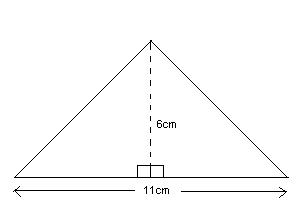Solution:

The area of a triangle may be found by using the formula, A=1/2bh, where brepresents the base and h represents the height. Thus, the area may be written as A=1/2(11)(6), or A = 33. The area of the triangle is 33 cm2.

QUESTION: 4

ABCD is an isosceles trapezium with lines AB parallel to CD.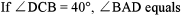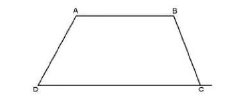Solution:

Isosceles trapezium is always cyclic The sum of opposite angles of a cyclic quadrilateral is 180°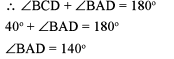QUESTION: 5

In the given figure, AB and CD are two chords of a circle intersecting at O. If AO = 4 cm and OB = 6 cm and OC = 3 cm. Find OD.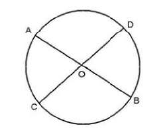Solution:

AO x OB = OC x OD

or, 4 x 6 = 3 x OD

OD = 8 CM

QUESTION: 6

In the above figure, O is the centre of the circle, and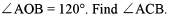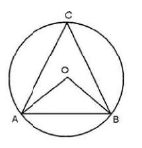Solution: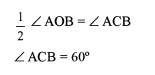The angle subtended by an arc of a circle at its center is twice of the angle it subtends anywhere on the circle’s circumference. The proof of this theorem is quite simple, and uses the exterior angle theorem – an exterior angle of a triangle is equal to the sum of the opposite interior angles. If the two opposite interior angles happen to be equal, then the exterior angle will be twice of any of the opposite interior angles.

QUESTION: 7

PQRS is a cyclic quadrilateral. If PQR is an equilateral triangle, find   ∠RSP. .

Solution: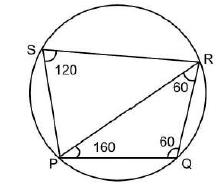QUESTION: 8

In a triangle ABC, the incentre is at O. If ∠BOC  = 100°, find ∠BAC.

Solution: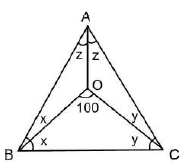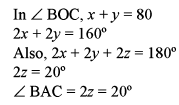QUESTION: 9

In the above figure, QR = 4 cm and RS = 12 cm. TS = 8 cm and QU is extended to T. Find PQ.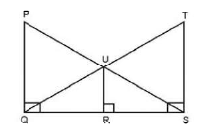Solution: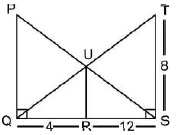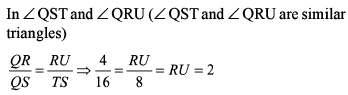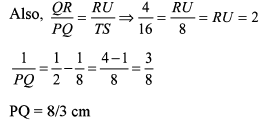QUESTION: 10

How many different regular polygons can be formed with the interior angle exceeding the exterior angle and the sum of the interior angles not exceeding 180°?

Solution:

Only 8 such regular polygons are possible.

QUESTION: 11

The ratio of the sides of Δ ABC is 1:2:4. What is the ratio of the altitudes drawn onto these sides?

Solution:

The triangle is not possible. So, (d) is the correct options

QUESTION: 12

The three sides of a triangle measure 6 cm, 8 cm and 10 cm respectively. A rectangle equal in area to the triangle has a length of 8 cm. The perimeter of the rectangle is:

Solution: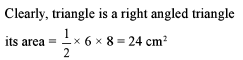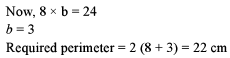QUESTION: 13

A rectangular enclosure 40 m x 36 m has a horse tethered to a corner with a rope of 14 m in length. What is the ratio of the respective areas it can graze, if it is outside the enclosure and if it is inside the enclosure?

Solution: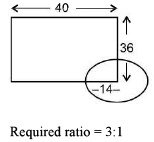QUESTION: 14

Read the passage below and solve the questions based on it The area of a square is equal lo the area of a rectangle. Moreover, the perimeter of the square is also equal to the perimeter of the rectangle,

Q.

The length of the rectangle is equal to the:

Solution:

This is possible only when both length and breadth of rectangle are equal to the side of the square.

QUESTION: 15

A quadrilateral is inscribed in a circle. If an angle is inscribed in each of the segments outside the quadrilateral, then what is the sum of the four angles?

Solution: